# DAV Class 4 Maths Chapter 4 Worksheet 2 Solutions

The DAV Class 4 Maths Book Solutions and DAV Class 4 Maths Chapter 4 Worksheet 2 Solutions of Division offer comprehensive answers to textbook questions.

## DAV Class 4 Maths Ch 4 WS 2 Solutions

Question 1.
Divide and write quotient and remainder,
(a) 7,525 ÷ 5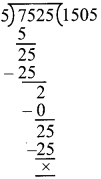Q = 1,505
R = 0

(b) 8,296 ÷ 4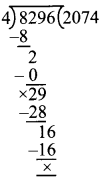Q = 2,074
R = 0

(c) 4,926 ÷ 7Q = 703
R = 4

(d) 2,786 ÷ 3Q = 928
R = 2

(e) 4,924 ÷ 8Q = 615
R = 4

(f) 4,528 ÷ 9Q = 503
R = 1

Question 2.
(a) 7,352 by 2Q = 3,676
R = 0
Divisor = 2
Dividend = 7,352

Dividend = Divisor × Q + R
L.H.S = 7,352
R.H.S = 2 × 3,676 + 0
R.H.S = 7,352
L.H.S = R.H.S

(b) 4,325 by 6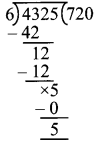Q = 720
R = 5
Divisor = 6
Dividend = 4,325
Dividend = Divisor × Q + R
L.H.S = 4,325
R.H.S = 720 × 6 + 5
R.H.S = 4,325
L.H.S = R.H.S
Hence, Checked(c) 7,316 by 5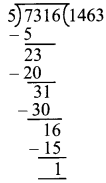Q = 1,463
R = 1
Divisor = 5
Dividend = 7,316
Dividend = Divisor × Q + R
L.H.S = 7,316
R.H.S = 1463 × 5 + 1
R.H.S = 7,316
L.H.S = R.H.S
Hence, Checked

(d) 4,217 by 8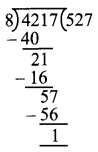Q = 527
R = 1
Divisor = 8
Dividend = 4,217

Dividend = Divisor × Q + R
L.H.S = 4,217
R.H.S = 8 × 527 + 1
= 4,217
L.H.S = R.H.S

Hence, checked

(e) 6,275 by 4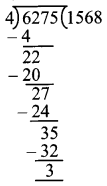Q = 1,568
R = 3
Divisor = 4
Dividend = 6,275

Dividend = Divisor × Q + R
L.H.S = 6,275
R.H.S = 4 × 1568 + 3
= 6,272 + 3
= 6,275
L.H.S = R.H.S

Hence, checked

(f) 1,026 by 3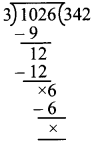Q = 342
R = 0
Divisor = 3
Dividend = 1,026

Dividend = Divisor × Q + R
L.H.S = 1,026
R.H.S = 3 × 342 + 0
= 1,026
L.H.S = R.H.S

Hence, checked

### DAV Class 4 Maths Chapter 4 Worksheet 2 Notes

Division (4-digit Number by single digit number):
Always remember that we stan division from the extreme left of dividend.
E.g. Divide 9,556 by 5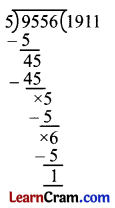Q = 1911
R = 1